Question

AP Mo G kb/4 k > The cantilevered beam shown here has a known rigidity, El,...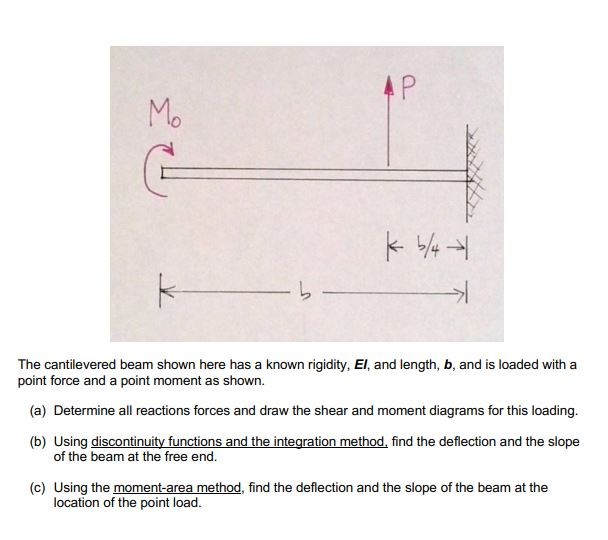AP Mo G kb/4 k > The cantilevered beam shown here has a known rigidity, El, and length, b, and is loaded with a point force and a point moment as shown. (a) Determine all reactions forces and draw the shear and moment diagrams for this loading. (b) Using discontinuity functions and the integration method, find the deflection and the slope of the beam at the free end. (c) Using the moment-area method, find the deflection and the slope of the beam at the location of the point load.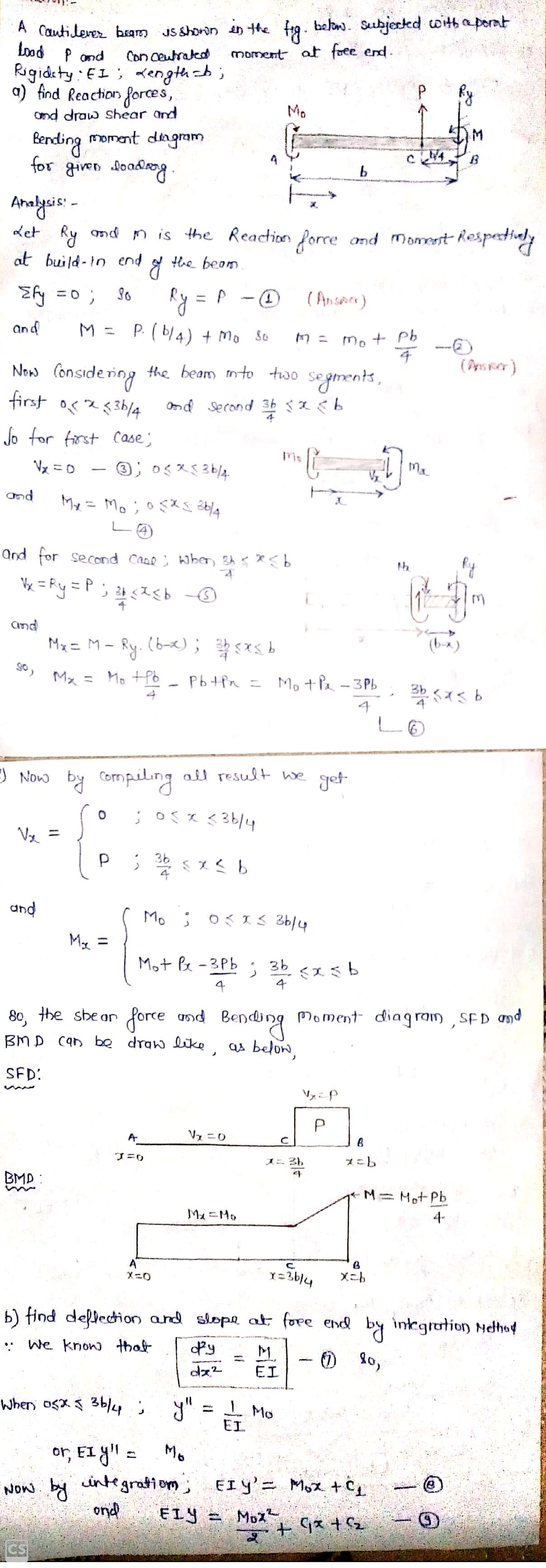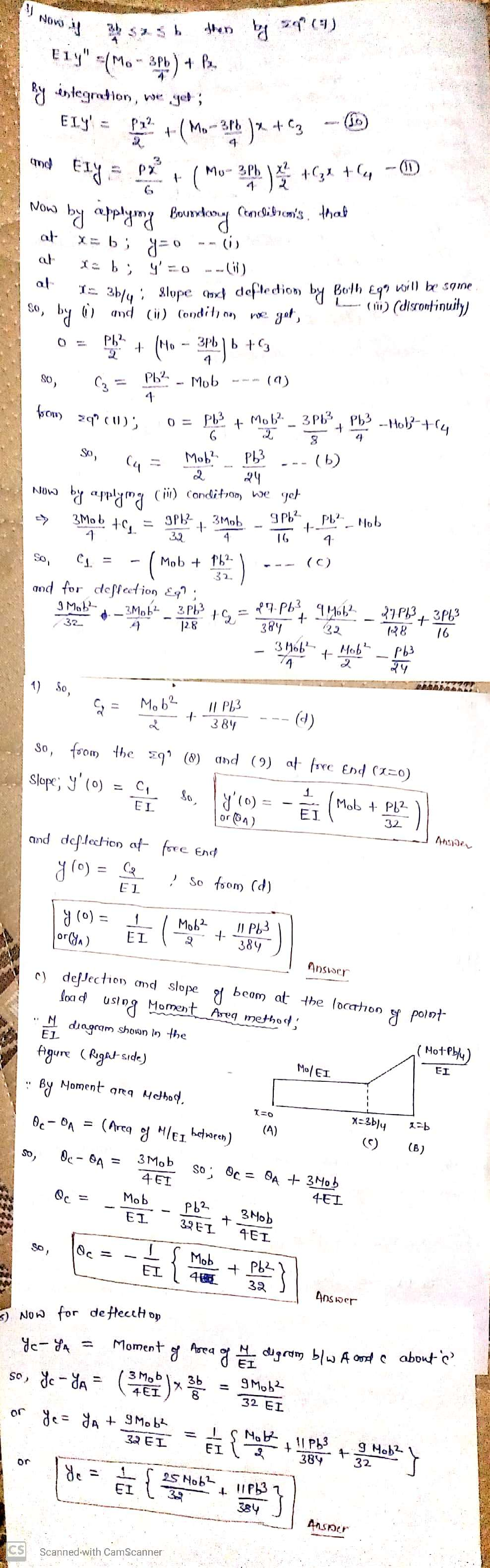Earn Coins

Coins can be redeemed for fabulous gifts.

Similar Homework Help Questions
• AP Mo kb/4 K The cantilevered beam shown here has a known rigidity, El, and length,...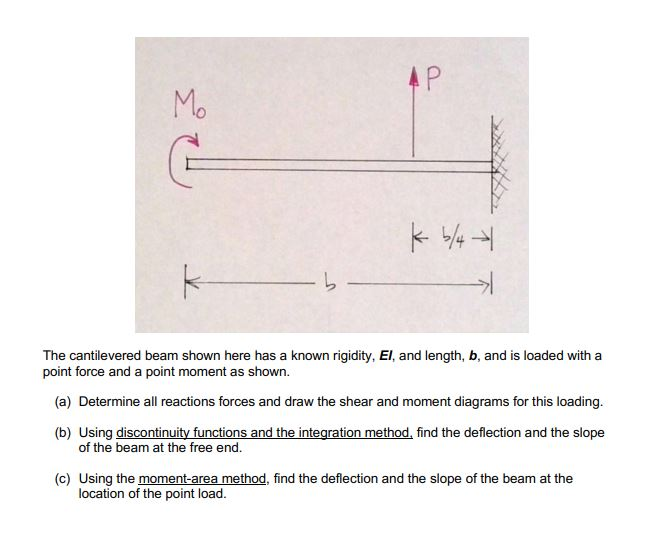AP Mo kb/4 K The cantilevered beam shown here has a known rigidity, El, and length, b, and is loaded with a point force and a point moment as shown. (a) Determine all reactions forces and draw the shear and moment diagrams for this loading. (b) Using discontinuity functions and the integration method, find the deflection and the slope of the beam at the free end. (c) Using the moment-area method, find the deflection and the slope of the beam...

• AP Mo k -> The cantilevered beam shown here has a known rigidity, El, and length,...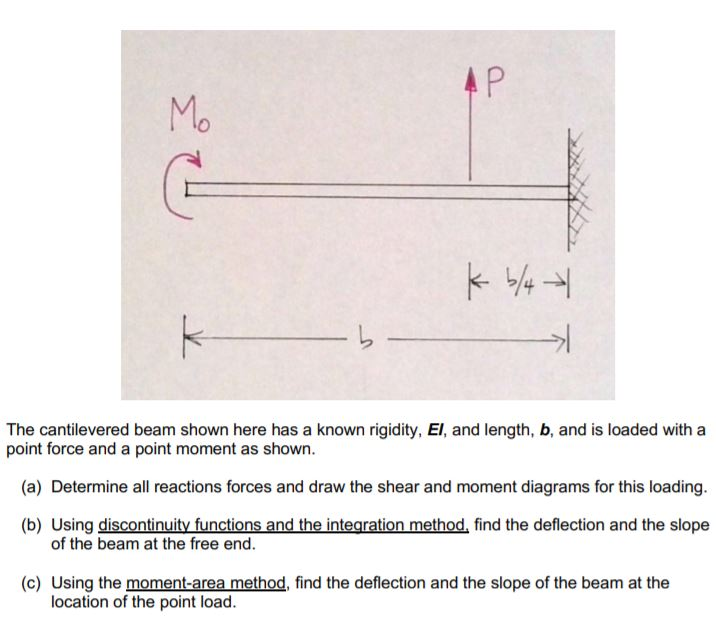AP Mo k -> The cantilevered beam shown here has a known rigidity, El, and length, b, and is loaded with a point force and a point moment as shown. (a) Determine all reactions forces and draw the shear and moment diagrams for this loading. (b) Using discontinuity functions and the integration method, find the deflection and the slope of the beam at the free end. (c) Using the moment-area method, find the deflection and the slope of the beam...

• The cantilevered beam shown here has a known rigidity, EI, and length, b, and is loaded...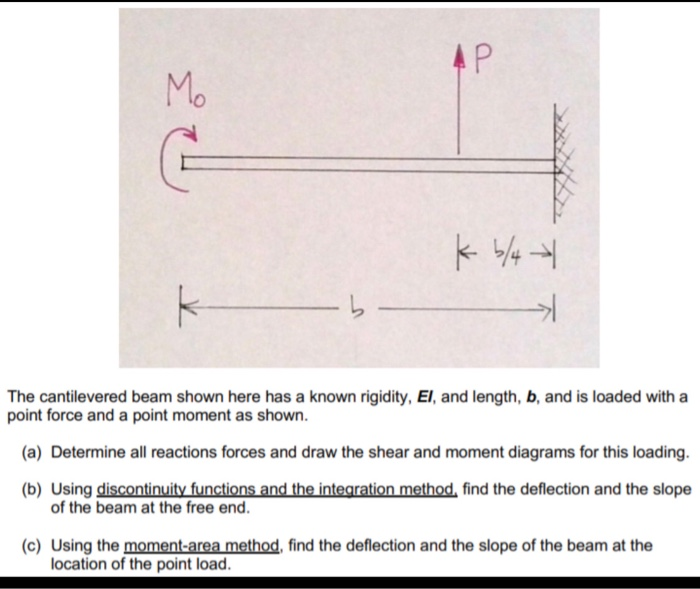The cantilevered beam shown here has a known rigidity, EI, and length, b, and is loaded with a point force and a point moment as shown a) Determine all reactions forces and draw the shear and moment diagrams for this loading. b) Using discontinuity functions and the integration method, find the deflection and the slope of the beam at the free end. c) Using the moment-area method, find the deflection and the slope of the beam at the location of...

• The cantilevered beam shown here has a known rigidity, EI, and length, b, and is loaded...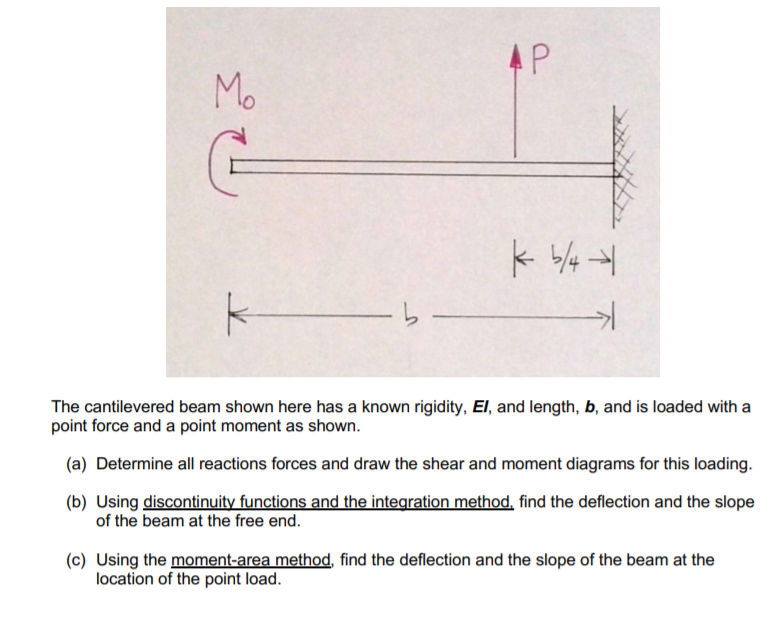The cantilevered beam shown here has a known rigidity, EI, and length, b, and is loaded with a point force and a point moment as shown. (a) Determine all reactions forces and draw the shear and moment diagrams for this loading. (b) Using discontinuity functions and the integration method, find the deflection and the slope of the beam at the free end. (c) Using the moment-area method, find the deflection and the slope of the beam at the location of...

• answers in terms of P,b and M AP Mo kb/4 k - The cantilevered beam shown...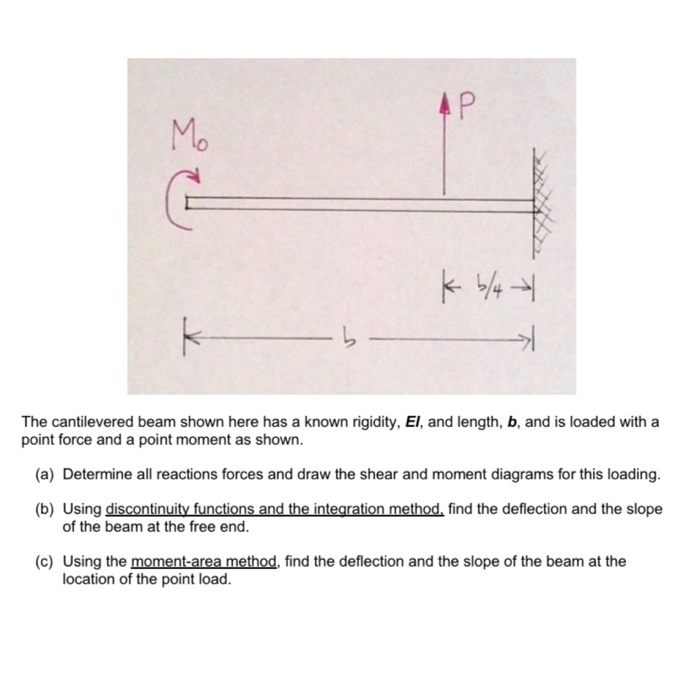answers in terms of P,b and M AP Mo kb/4 k - The cantilevered beam shown here has a known rigidity, El, and length, b, and is loaded with a point force and a point moment as shown. (a) Determine all reactions forces and draw the shear and moment diagrams for this loading. (b) Using discontinuity functions and the integration method, find the deflection and the slope of the beam at the free end. (c) Using the moment-area method, find...

• A simply supported uniform beam (with length L and flexural rigidity El) carries a moment Mo...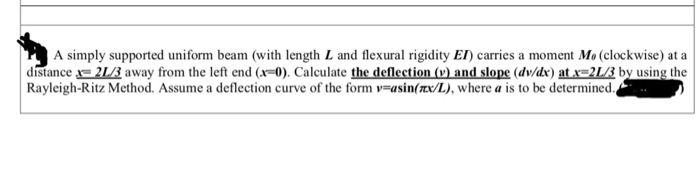A simply supported uniform beam (with length L and flexural rigidity El) carries a moment Mo (clockwise) at a distance -21B away from the left end (x-0). Calculate the deflection () and slope (dv/de) at 21/3 by using the Rayleigh-Ritz Method. Assume a deflection curve of the form v-asin(rx/L), where a is to be determined

• slope and deflection please Consider the beam and loading shown. Assume that the flexural rigidity El...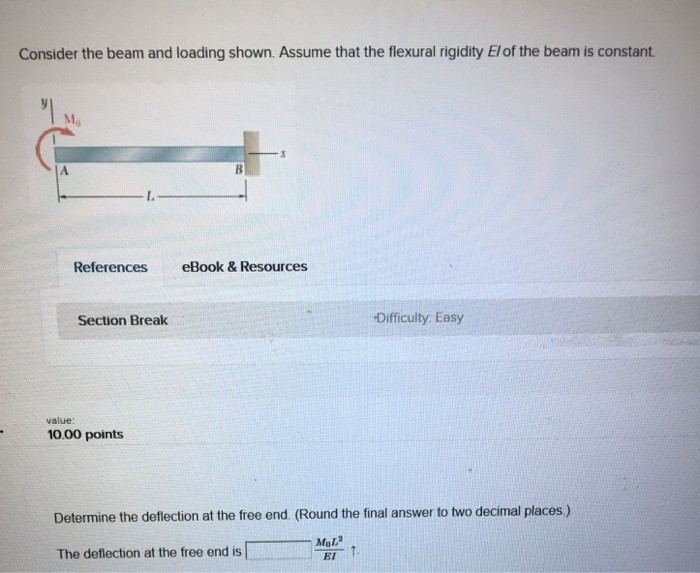slope and deflection please Consider the beam and loading shown. Assume that the flexural rigidity El of the beam is constant References eBook & Resources Section Break -Difficulty: Easy value: 10.00 points Determine the deflection at the free end. (Round the final answer to two decimal places.) The deflection at the free end is

• For the loading shown in the below figure, knowing that wo 2 kN/m, the length of the beam is L 2 ...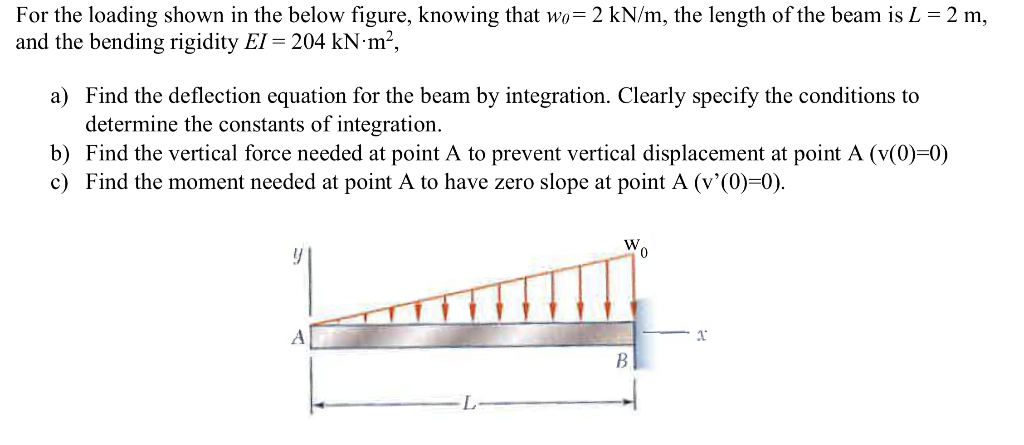For the loading shown in the below figure, knowing that wo 2 kN/m, the length of the beam is L 2 m, and the bending rigidity EI-204 kN-m2, a) Find the deflection equation for the beam by integration. Clearly specify the conditions to determine the constants of integration b) Find the vertical force needed at point A to prevent vertical displacement at point A (v(0)-0) c) Find the moment needed at point A to have zero slope at point A...

• For the cantilever beam with a constant El and loading shown, using the superposition method to...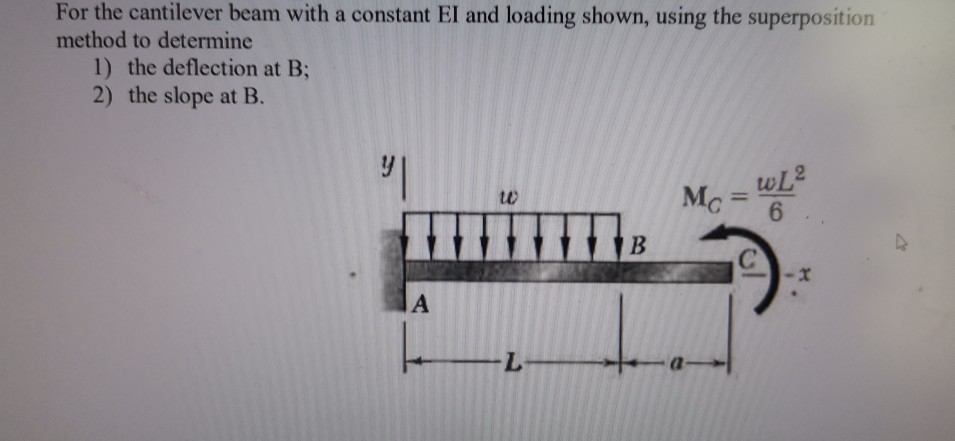For the cantilever beam with a constant El and loading shown, using the superposition method to determine 1) the deflection at B; 2) the slope at B. MWL Mo= 6

• Consider a cantilever beam under a concentrated force and moment as shown below. The deflections ofthe...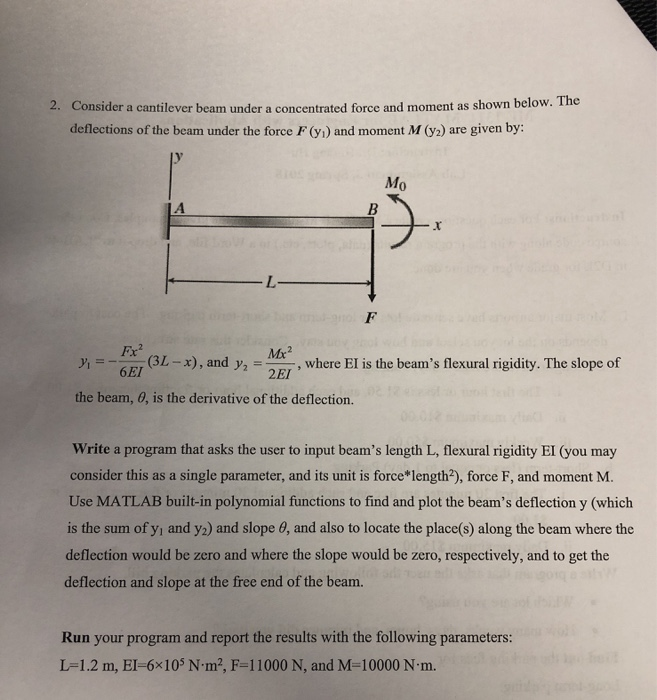Consider a cantilever beam under a concentrated force and moment as shown below. The deflections ofthe beam under the force F (y) and moment M (y) are given by: 2. y' Mo L-x) , and y2 Me , where EI is the beam's flexural rigidity. The slope of the beam, 0, is the derivative of the deflection. Write a program that asks the user to input beam's length L, flexural rigidity EI (you may consider this as a single parameter,...